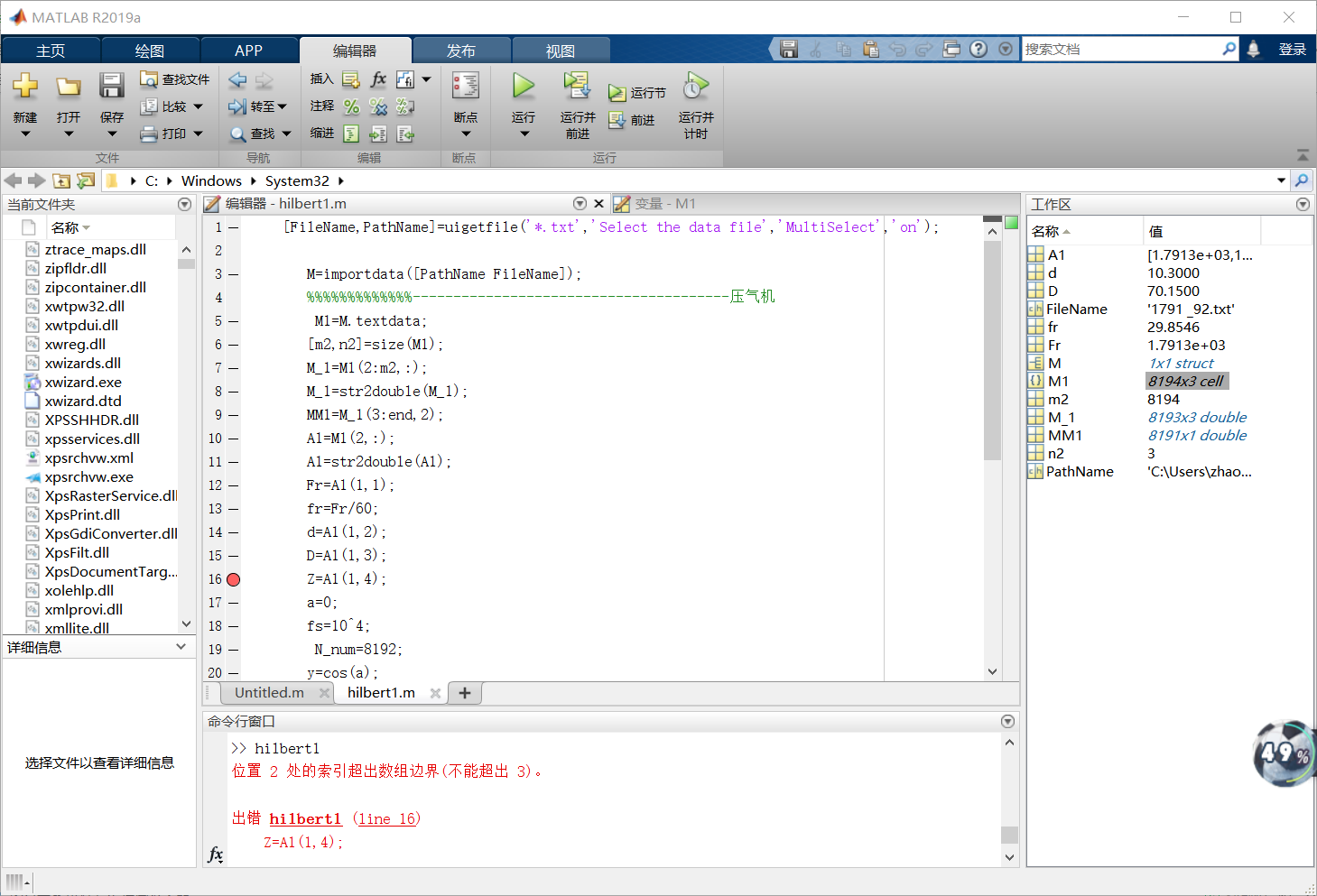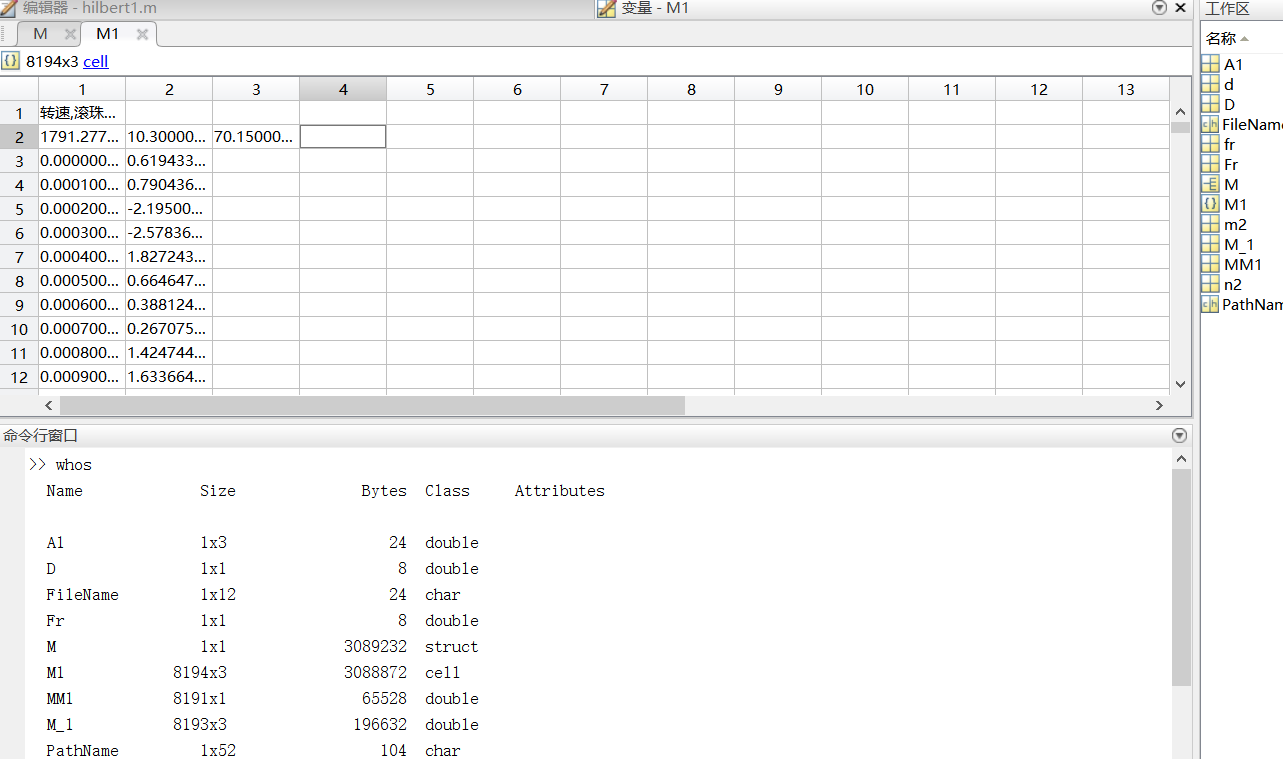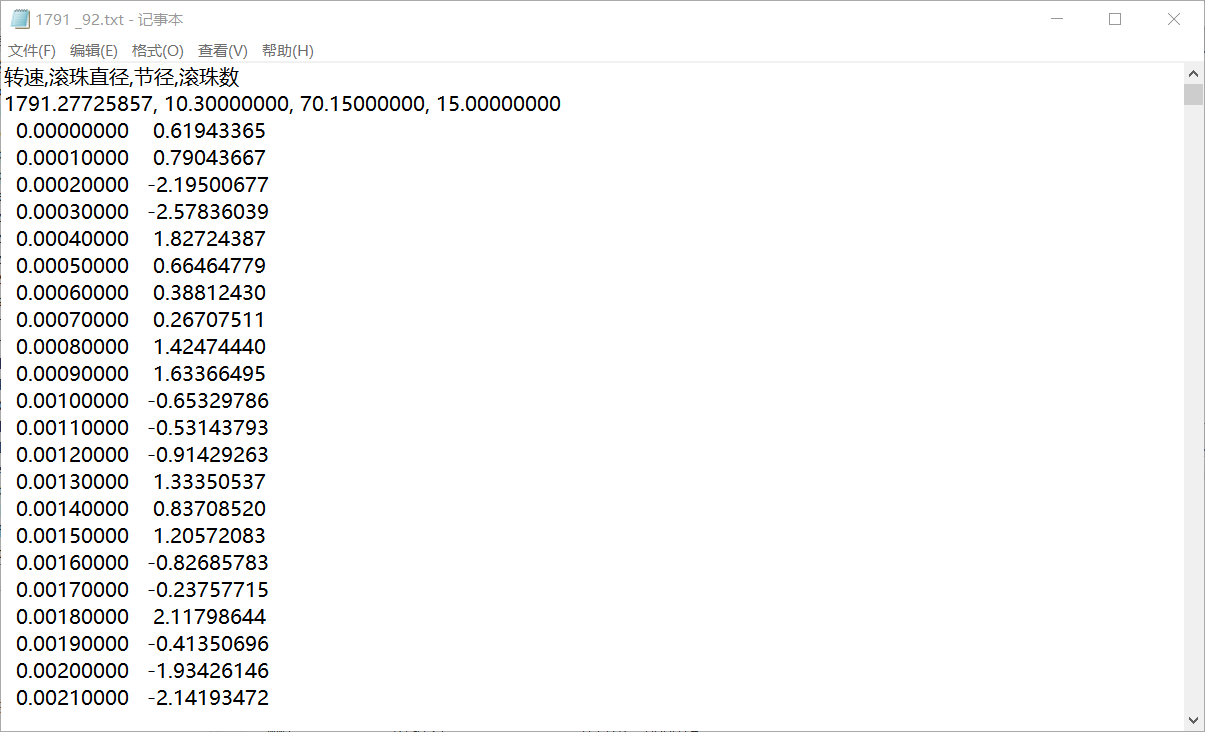2019-07-27 19:38

# 用importdata函数把数据从txt导入matlab后 少了一列

``````[FileName,PathName]=uigetfile('*.txt','Select the data file','MultiSelect','on');

M=importdata([PathName FileName]);
%%%%%%%%%%%%%---------------------------------------压气机
M1=M.textdata;
[m2,n2]=size(M1);
M_1=M1(2:m2,:);
M_1=str2double(M_1);
MM1=M_1(3:end,2);
A1=M1(2,:);
A1=str2double(A1);
Fr=A1(1,1);
fr=Fr/60;
d=A1(1,2);
D=A1(1,3);
Z=A1(1,4);
``````• 点赞
• 写回答
• 关注问题
• 收藏
• 复制链接分享
• 邀请回答

#### 2条回答

• 这样和你说，比如说你的txt文件叫a.txt。
你把a.txt拖到你的工作区里
输入a=importdata('a.txt');
没用你来砍我

点赞 2 评论 复制链接分享
• 额能因为设置下标不对

点赞 1 评论 复制链接分享